Next: Dirac Delta Function Up: Maxwell's Equations Previous: Maxwell's Equations

# Scalar and Vector Potentials

We can automatically satisfy Equation (2) by writing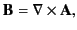(11)

where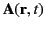is termed the vector potential. Furthermore, we can automatically satisfy Equation (3) by writing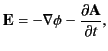(12)

where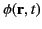is termed the scalar potential.

The previous prescription for expressing electric and magnetic fields in terms of the scalar and vector potentials does not uniquely define the potentials. Indeed, it can be seen that if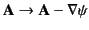and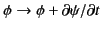, where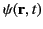is an arbitrary scalar field, then the associated electric and magnetic fields are unaffected. The root of the problem lies in the fact that Equation (11) specifies the curl of the vector potential, but leaves the divergence of this vector field completely unspecified. We can make our prescription unique by adopting a convention that specifies the divergence of the vector potential--such a convention is usually called a gauge condition. It turns out that Maxwell's equations are Lorentz invariant. (See Chapter 12.) In other words, they take the same form in all inertial frames. Thus, it makes sense to adopt a gauge condition that is also Lorentz invariant. This leads us to the so-called Lorenz gauge condition (see Section 12.12),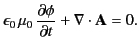(13)

Equations (11)-(13) can be combined with Equations (1) and (4) to give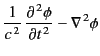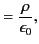(14)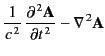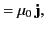(15)

where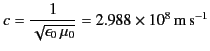(16)

is the velocity of light in vacuum. Thus, Maxwell's equations essentially boil down to Equations (14) and (15).Next: Dirac Delta Function Up: Maxwell's Equations Previous: Maxwell's Equations
Richard Fitzpatrick 2014-06-27